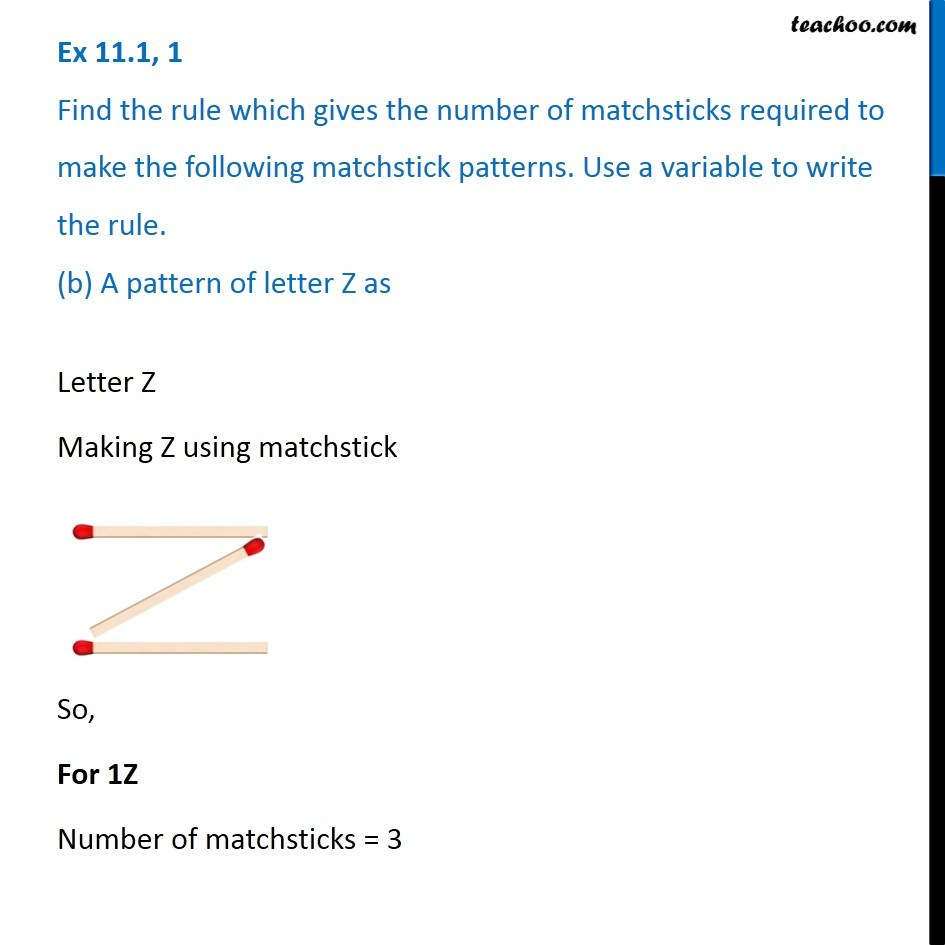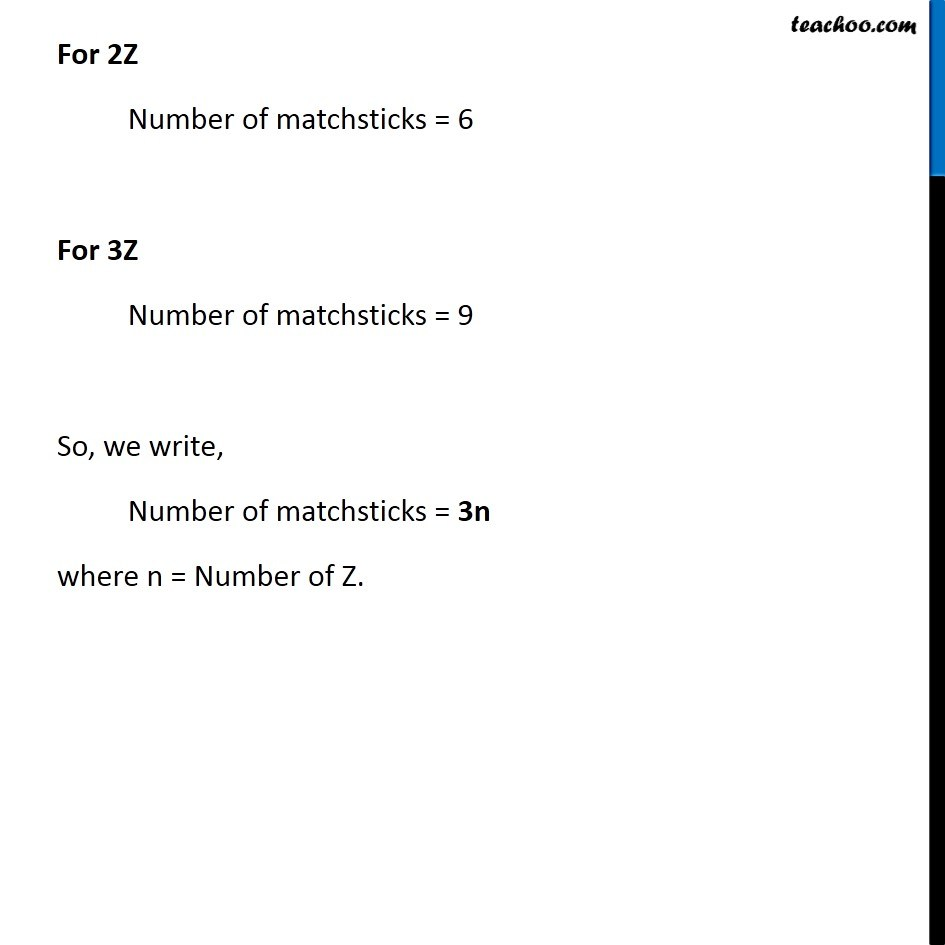Ex 11.1

Chapter 11 Class 6 Algebra
Serial order wiseGet live Maths 1-on-1 Classs - Class 6 to 12

### Transcript

Ex 11.1, 1 Find the rule which gives the number of matchsticks required to make the following matchstick patterns. Use a variable to write the rule. (b) A pattern of letter Z asLetter Z Making Z using matchstick So, For 1Z Number of matchsticks = 3 For 2Z Number of matchsticks = 6 For 3Z Number of matchsticks = 9 So, we write, Number of matchsticks = 3n where n = Number of Z.